Definitions of Square Dance Calls and Concepts

Index -->  Plus  |  A1  |  A2  |  C1  |  C2  |  C3A  |  C3B  |  C4  |  NOL  |
Definitions (Text Only) -->  Plus  |  A1  |  A2  |  C1  |  C2  |  C3A  |  C3B  |  C4  |  NOL  |
 Find call:

\$BJ?9T\$J(B Wave \$B\$+\$i!%(B

Leaders \$B\$,(B 1/2 Split Circulate \$B\$r\$7\$F(B Tandem Cross Fold \$B\$r\$9\$k4V\$K(B, Trailers \$B\$O(B Follow Your Neighbor (Extend and Arm Turn 3/4) \$B\$r\$7(B, \$BA40w\$G(B Tandem Extend \$B\$r\$7\$^\$9!%(B

Column \$B\$G=*\$o\$j\$^\$9!%(B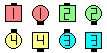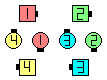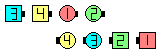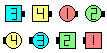Follow Your Leader\$B\$NA0(B 1/2 Split Circulate\$B\$N8e(B Outsides Tandem Cross Fold as Centers Arm Turn 3/4\$B\$N8e(B Tandem Extend\$B\$N8e(B (\$B=*\$o\$j(B)

Your Leader [C3A]: \$B1&\$N(B 3/4 Tag \$B\$+\$i!%(B Centers \$B\$,(B Arm Turn 3/4 \$B\$r(B, \$BB>\$N?M\$O(B 1/4 Right \$B\$r\$7\$F(B Tandem Cross Fold \$B\$r\$7(B, \$BA40w\$G(B Tandem Extend \$B\$r\$7\$^\$9!%(B Column \$B\$G=*\$o\$j\$^\$9!%(B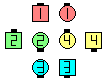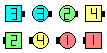Your Leader\$B\$NA0(B \$B8e(B

\$BCmYour Leader \$B\$O(B Twin Diamonds \$B\$+\$i\$b%3!<%k\$5\$l\$F\$*\$j(B, \$B\$3\$N>l9g(B Outsides \$B\$O(B 1/4 Right \$B\$r>JN,\$7\$^\$9!%\$3\$N;H\$\$J}\$O(B, \$B:#\$O(B '\$B\$(!

\$B%@%s%9\$N%R%s%H(B: Arm Turn 3/4 \$B\$r\$9\$k?M\$O(B, Tandem Extend \$B\$r\$9\$kA0\$KBT\$DI,MW\$,\$"\$k>l9g\$,\$"\$j\$^\$9!%(BOutsides \$B\$,<+J,\$N%Q!<%H\$r\$9\$k\$N\$K;~4V\$,\$+\$+\$k\$3\$H\$,\$"\$k\$+\$i\$G\$9!%(B

\$BTagging Calls.CALLERLAB definition for Follow Your LeaderChoreography for Follow Your LeaderComments? Questions? Suggestions?